Next: Quadrupole moments Up: Superdeformed bands in S Previous: Superdeformed bands in S

## Energies

In Fig. 6 are plotted the HF energies as functions of spin for the 30 SD bands calculated in 32S. As it is often done in the cranking approach, we identify the average projection of the angular momentum on the cranking axis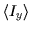with the total angular momentum of the system, i.e., we set I=. (Within a more refined approximation some authors identify I(I+1) with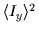, what results in a standard (approximate) correction I=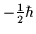; however, this is not implemented in the figures presented below.)

All the bands have been obtained within the cranking HF formalism, with the rotational frequencies that start at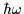=0.4MeV and increase in steps of 0.2MeV. For each band, the calculations were carried out up to the highest rotational frequencies that did not induce any sudden configuration change. Since almost all bands are crossed at high rotational frequencies by the bands involving the down-sloping 1/2(r=-i) orbital, cf. Figs. 1 and 2, and Ref. , such configuration changes are in many cases inevitable. On the one hand, introducing an upper limit of the frequencies of some calculated bands reflects a deficiency of the method since the discussed crossings are in general the physical ones. On the other hand, however, the corresponding experimental results are expected to deviate from regularity at the vicinity of the calculated limitingvalues and are likely to manifest, e.g., an up- or even a back-bending behavior there, thus offering a possibility of valuable tests of the crossing frequencies anyway.

Let us remark in passing that within the HO model, when two protons and two neutrons in the HO 1/2 states are added to the 32S SD configuration, one obtains the magic hyperdeformed HO configuration in 36Ar. Structures based on the 1/2(r=-i) orbitals are abundant in 32S, however, they should rather be attributed to the hyperdeformed configurations, and are not studied in the present article.

Bands shown in Fig. 6 have been separated into four groups, plotted in four panels. Figures 6(a), (b), (c), and (d) show the 3n3p bands with p=n, p=n+1, p=n+2, and p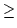n+3, respectively. Parities of bands are equal to the products of parities of the proton and neutron configurations, i.e.,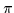= (-1)n+p in our case, and are denoted by full (=+1) and open (=-1) symbols. Various forms of the symbols (circles, squares, etc.) distinguish different values of n. In order to further differentiate between various configurations, we have to introduce a convention relating the line styles with the signatures of neutron and proton subsystems, rn and rp. Hence, long-short-dashed, solid, dotted, and dashed lines denote (rn,rp)=(+,+), (+,-), (-,+), and (-,-) signatures, respectively. Of course the total signature rof each band is always equal to r=rn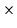rp.

Since we are mostly interested in the low energy configurations, in Fig. 7 we show a blow-up of the near-yrast region of energies, for a selection of bands being closest to the yrast band. At I=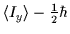= 6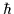(with the standard spin correction of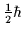here subtracted), we obtain in the 3030 band the total energy of E=-261.651MeV, which gives the calculated excitation energy of Ex(I=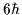)=8.349MeV, ridiculously close to the experimental energy, 8.346MeV [31,32], of the 6+ yrast state in 32S. Of course, an agreement on this level of precision is to a large extent accidental, however, it gives us confidence that a correct configuration is being followed at low excitation energies.

At low spins, the yrast line is first built upon the ground-state 3030 configuration whose energy increases very regularly up to the angular momentum of I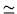9and excitation energy of Ex14.5MeV. At I9-10, the one-intruder configurations 3031 become yrast for a narrow region of spins. These bands are next crossed at Ex16.2MeV by two bands with r=+1, the 3131 configurations, which are yrast up to about I15. At this point (Ex25MeV) the yrast line has the structure of the magic 3232 SD configuration. When extrapolated to zero spin, the magic SD configuration corresponds to the excitation energy of Ex14MeV.

The spin (energy) range of up to I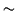6(10MeV) can be very well described by the sd shell-model calculations , and in the rest of this article we will focus on the higher spin states. [Some collective bands in the low spin (energy) range may be unstable with respect to parity-breaking deformations ; we do not study those effects either.]Next: Quadrupole moments Up: Superdeformed bands in S Previous: Superdeformed bands in S
Jacek Dobaczewski
1999-07-27